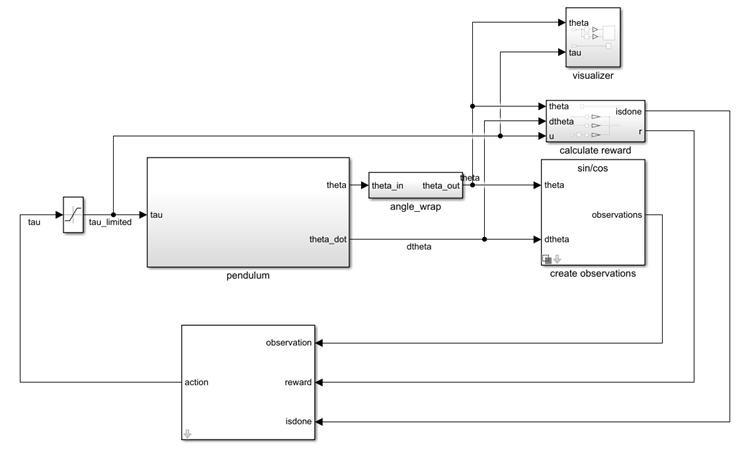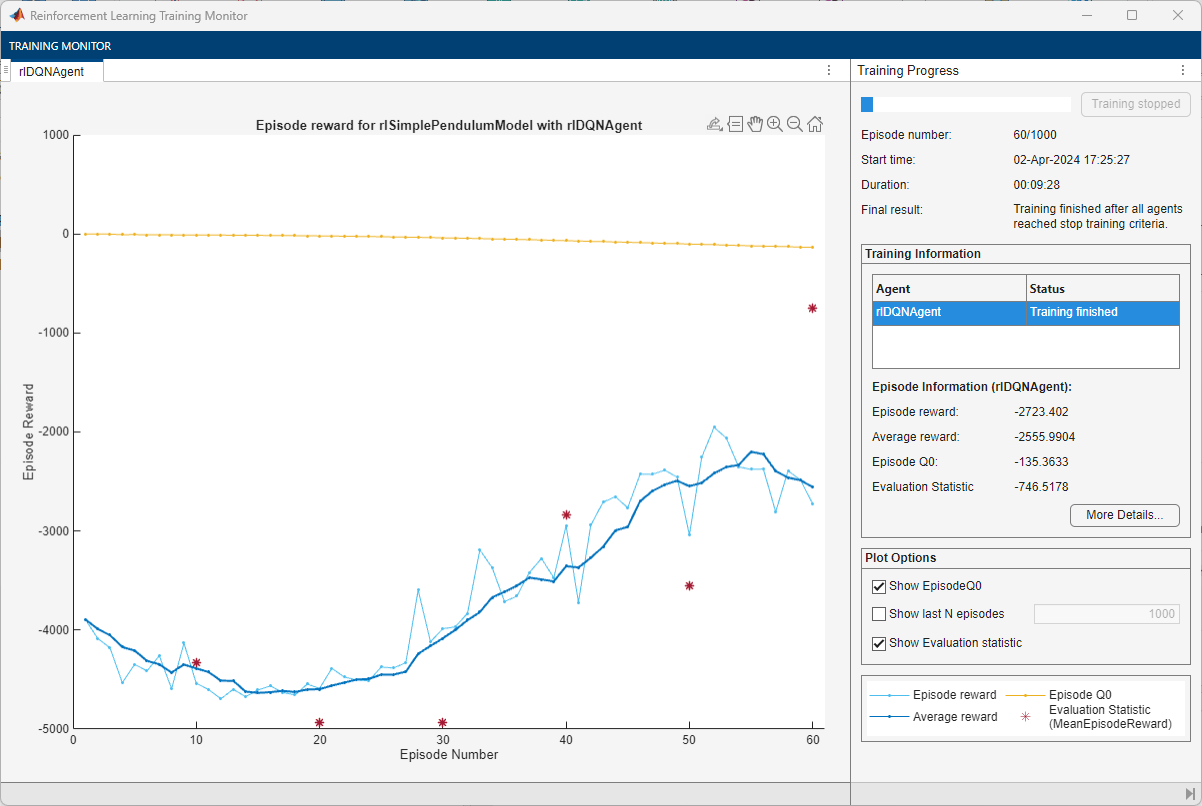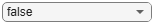# Train DQN Agent to Swing Up and Balance Pendulum

This example shows how to train a deep Q-learning network (DQN) agent to swing up and balance a pendulum modeled in Simulink®.

For more information on DQN agents, see Deep Q-Network (DQN) Agents. For an example that trains a DQN agent in MATLAB®, see Train DQN Agent to Balance Cart-Pole System.

### Pendulum Swing-up Model

The reinforcement learning environment for this example is a simple frictionless pendulum that initially hangs in a downward position. The training goal is to make the pendulum stand upright without falling over using minimal control effort.

Open the model.

```mdl = 'rlSimplePendulumModel'; open_system(mdl)```For this model:

• The upward balanced pendulum position is 0 radians, and the downward hanging position is `pi` radians.

• The torque action signal from the agent to the environment is from –2 to 2 N·m.

• The observations from the environment are the sine of the pendulum angle, the cosine of the pendulum angle, and the pendulum angle derivative.

• The reward ${\mathit{r}}_{\mathit{t}}$, provided at every timestep, is

`${\mathit{r}}_{\mathit{t}}=-\left({{\theta }_{\mathit{t}}}^{2}+0.1{\stackrel{˙}{{\theta }_{\mathit{t}}}}^{2}+0.001{\mathit{u}}_{\mathit{t}-1}^{2}\right)$`

Here:

• ${\theta }_{\mathit{t}}$ is the angle of displacement from the upright position.

• $\stackrel{˙}{{\theta }_{\mathit{t}}}$ is the derivative of the displacement angle.

• ${\mathit{u}}_{\mathit{t}-1}$ is the control effort from the previous time step.

### Create Environment Interface

Create a predefined environment interface for the pendulum.

`env = rlPredefinedEnv('SimplePendulumModel-Discrete')`
```env = SimulinkEnvWithAgent with properties: Model : rlSimplePendulumModel AgentBlock : rlSimplePendulumModel/RL Agent ResetFcn : [] UseFastRestart : on ```

The interface has a discrete action space where the agent can apply one of three possible torque values to the pendulum: –2, 0, or 2 N·m.

To define the initial condition of the pendulum as hanging downward, specify an environment reset function using an anonymous function handle. This reset function sets the model workspace variable `theta0` to `pi`.

`env.ResetFcn = @(in)setVariable(in,'theta0',pi,'Workspace',mdl);`

Get the observation and action specification information from the environment

`obsInfo = getObservationInfo(env)`
```obsInfo = rlNumericSpec with properties: LowerLimit: -Inf UpperLimit: Inf Name: "observations" Description: [0x0 string] Dimension: [3 1] DataType: "double" ```
`actInfo = getActionInfo(env)`
```actInfo = rlFiniteSetSpec with properties: Elements: [3x1 double] Name: "torque" Description: [0x0 string] Dimension: [1 1] DataType: "double" ```

Specify the simulation time `Tf` and the agent sample time `Ts` in seconds.

```Ts = 0.05; Tf = 20;```

Fix the random generator seed for reproducibility.

`rng(0)`

### Create DQN Agent

A DQN agent approximates the long-term reward, given observations and actions, using a value function critic.

Since DQN has a discrete action space, it can rely on a multi-output critic approximator, which is generally a more efficient option than relying on a comparable single-output approximator. A multi-output approximator has only the observation as input and an output vector having as many elements as the number of possible discrete actions. Each output element represents the expected cumulative long-term reward following from the observation given as input, when the corresponding discrete action is taken.

To create the critic, first create a deep neural network with an input vector of three elements ( for the sine, cosine, and derivative of the pendulum angle) and one output vector with three elements (–2, 0, or 2 Nm actions). For more information on creating a deep neural network value function representation, see Create Policies and Value Functions.

```dnn = [ featureInputLayer(3,'Normalization','none','Name','state') fullyConnectedLayer(24,'Name','CriticStateFC1') reluLayer('Name','CriticRelu1') fullyConnectedLayer(48,'Name','CriticStateFC2') reluLayer('Name','CriticCommonRelu') fullyConnectedLayer(3,'Name','output')]; dnn = dlnetwork(dnn);```

View the critic network configuration.

```figure plot(layerGraph(dnn))```Specify options for the critic optimizer using `rlOptimizerOptions`.

`criticOpts = rlOptimizerOptions('LearnRate',0.001,'GradientThreshold',1);`

Create the critic representation using the specified deep neural network and options. You must also specify observation and action info for the critic. For more information, see `rlVectorQValueFunction`.

`critic = rlVectorQValueFunction(dnn,obsInfo,actInfo);`

To create the DQN agent, first specify the DQN agent options using `rlDQNAgentOptions`.

```agentOptions = rlDQNAgentOptions(... 'SampleTime',Ts,... 'CriticOptimizerOptions',criticOpts,... 'ExperienceBufferLength',3000,... 'UseDoubleDQN',false);```

Then, create the DQN agent using the specified critic representation and agent options. For more information, see `rlDQNAgent`.

`agent = rlDQNAgent(critic,agentOptions);`

### Train Agent

To train the agent, first specify the training options. For this example, use the following options.

• Run each training for at most 1000 episodes, with each episode lasting at most `500` time steps.

• Display the training progress in the Episode Manager dialog box (set the `Plots` option) and disable the command line display (set the `Verbose` option to `false`).

• Stop training when the agent receives an average cumulative reward greater than –1100 over five consecutive episodes. At this point, the agent can quickly balance the pendulum in the upright position using minimal control effort.

• Save a copy of the agent for each episode where the cumulative reward is greater than –1100.

For more information, see `rlTrainingOptions`.

```trainingOptions = rlTrainingOptions(... 'MaxEpisodes',1000,... 'MaxStepsPerEpisode',500,... 'ScoreAveragingWindowLength',5,... 'Verbose',false,... 'Plots','training-progress',... 'StopTrainingCriteria','AverageReward',... 'StopTrainingValue',-1100,... 'SaveAgentCriteria','EpisodeReward',... 'SaveAgentValue',-1100);```

Train the agent using the `train` function. Training this agent is a computationally intensive process that takes several minutes to complete. To save time while running this example, load a pretrained agent by setting `doTraining` to `false`. To train the agent yourself, set `doTraining` to `true`.

```doTraining = false; if doTraining % Train the agent. trainingStats = train(agent,env,trainingOptions); else % Load the pretrained agent for the example. load('SimulinkPendulumDQNMulti.mat','agent'); end```### Simulate DQN Agent

To validate the performance of the trained agent, simulate it within the pendulum environment. For more information on agent simulation, see `rlSimulationOptions` and `sim`.

```simOptions = rlSimulationOptions('MaxSteps',500); experience = sim(env,agent,simOptions);```